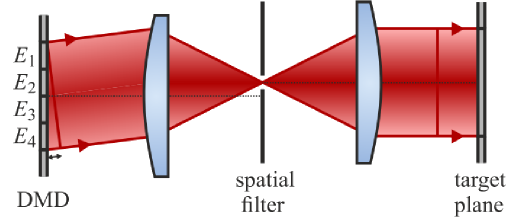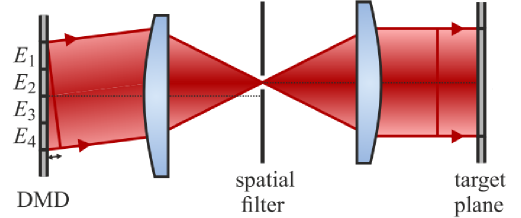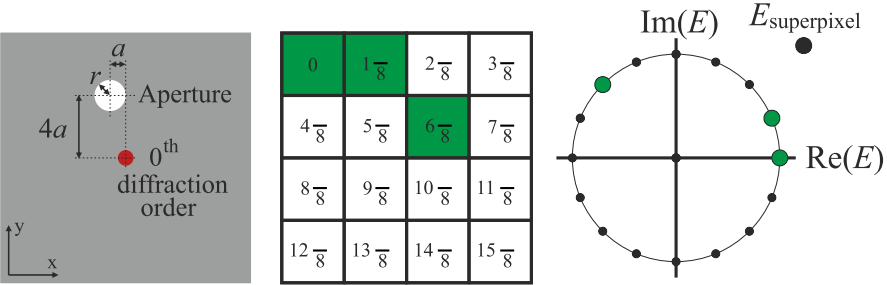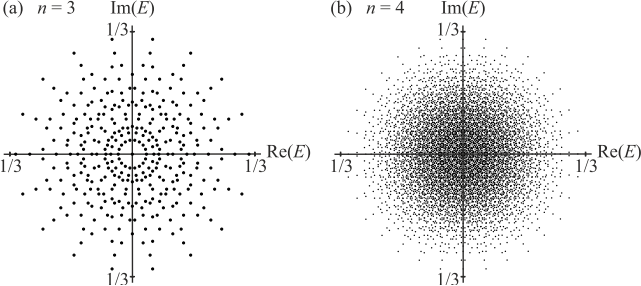## How to use a binary amplitude Deformable Miror Device (DMD) as a phase modulator: The "superpixel" methodI previously presented a technique based on the Lee hologram that allows to use a binary amplitude modulator (like a DLP chip you find in standard projectors) to perform a phase modulation (or amplitude and phase modulation). Recently, a new technique was introduced in [S.A. Goorden et al., Opt. Express (2014)] that allows an accurate complex modulation with less loss in term of spatial resolution. This post is more a highlight on this paper than a proper tutorial. In a nutshell, while the Lee hologram only takes advantage of one dimension to encode the amplitude and phase in fringes, this technique exploits both dimensions of the pixel array using superpixels.

The basic idea is to use $$n\times n$$ superpixels. The image is filtered in the Fourier plane so that individual pixels cannot be resolved and the final image of one superpixel is approximately the sum of the contributions of all the pixels. The key is to have the phase of the n² pixels that are homogeneously distributed between 0 and 2π. Then, by switching on or off pixels inside a superpixel, we create a suitable combination that approximates the desired field.Figure 1. Schematic of the setup. Image from [S.A. Goorden et al., Opt. Express (2014)].

Let's go back to the setup. To obtain the desired phase contributions for each pixel, one put a mask with a circular aperture in the Fourier plane. The size of the disk is chosen to filter out the frequencies corresponding to the repetition of the individual pixels but not (too much) the frequencies corresponding to the signal of the desired image. In other words, the goal is to average the effect of the individual pixels in one superpixel but not to average two successive superpixels. The position of the aperture is chosen at $$(x,y)=(a,-na)$$ where $$a=\frac{-\lambda f}{n^2 d}$$, $$\lambda$$ is the wavelength, $$f$$ the focal length of the first lens and $$d$$ the pitch of the pixel array. This way, the phase difference in the contributions of two neighboring pixels in the x-direction is $$\frac{2\pi}{n^2}$$ and $$\frac{2\pi}{n}$$ in the y-direction.Figure 2. Schematic of the Fourier plane of the 4-f system (left). Relative phase contributions of pixels inside a 4x4 superpixel (center). Polar representation of those contributions (right). Image from [S.A. Goorden et al., Opt. Express (2014)].

If we neglect the fact that the pixels of one superpixel do not exactly everlap spatially (they are close but not at the same place, which is not possible) the complex field given by one superpixel is the mathematical sum of all the pixel contributions. Be careful! This assertion is quite wrong in the general case. If the image to display has very sharp patterns, the image can be significalty blurred. This is due to the fact that the high spatial frequencies of the image are filtered out by the mask we use to remove repetition spatial frequency of the pixels. In other words, the diameter size of the mask we chose not to see the small pixels independently has the effect to blur the final image.

In figure 2, the authors show an example of three pixels switched on in a 4x4 superpixel and the resulting field in the polar representation. This creates a great variety of possibilities even for a small superpixel size n.Figure 3. Constellation diagram representing the complex fields possible to obtain with a 3x3 (a) and a 4x4 (b) superpixel. Image from [S.A. Goorden et al., Opt. Express (2014)].

Figure 3 shows the constellation of complex fields achievable for n = 3 and n = 4. As important as the number of combinations possible, we notice that it is possible to obtain a fair amount of possibilities that are approximately located on a circle. That means that one can use this technique to do phase-only modulation with a reasonable amount a phase values possible. To approximate a given desired complex field, we chose the combination which is the closest in the complex plane, and switch on the corresponding combination of pixels in the superpixel.

As a rule of thumb, the number of possible combinations possible is linked to the number of independent pixels one can control to generate the complex field in one segment on the final image. In the Lee hologram technique, if the diameter size of the mask in the Fourier plane is set to average roughly 16 neighboring pixels, we can control 16 pixels values for one segment as we have fringes in one direction. With the superpixel technique, we make use of all the 256 pixels in a 16x16 square. This argument is very qualitative, I plan to make simulations to compare both techniques.

Created by sebastien.popoff on 24/10/2014